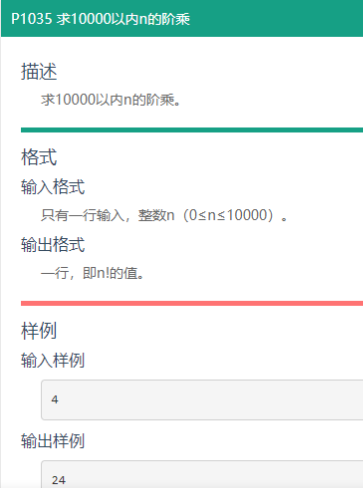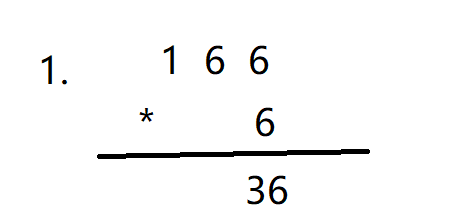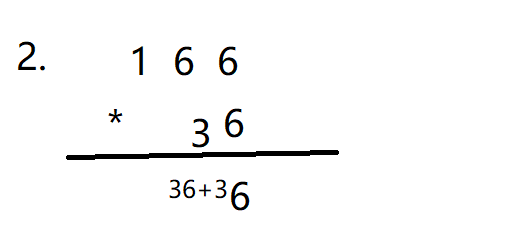# 一、题目# 三、如何将大数存储在数组里首先是66得到36，因为是十进制，所以一位的大小要小于十，所以就发生了进位。# 代码的实现

`for (int i = 1; i <= n; i++)     {         for (int j = 0; j < 10000; j++)         {             arr[j] = arr[j] * i + b;             b=arr[j]/10;//要进位的数             arr[j] = arr[j] % 10;         }     }`

# 整体逻辑的实现

`include<stdio.h> #include<string.h> #pragma warning(disable:4996) int main() {     int n;     scanf("%d", &n);     if(n==0)     {     printf("0");     return 0;     }     int arr = { 0 };      arr = 1;     int a = n;     int b = 0;     int c = 0;     for (int i = 1; i <= n; i++)     {         for (int j = 0; j < 10000; j++)         {             arr[j] = arr[j] * i + b;             b=arr[j]/10;//要进位的数             arr[j] = arr[j] % 10;         }     }     int size = 9999;     while (arr[size] == 0)     {         size--;     }     for (int i = size; i >= 0; i--)     {         printf("%d", arr[i]);     }     return 0; }`

# 存在的问题和反思

1.在一开始的时候，数组没有初始化，导致在寻找首数字的时候出错了，以后一定要记得初始化数组

2.记得要考虑特殊情况，（n==0）的时候。

3.一定要缕清楚整个进位的过程和方法。

4.while(arr[size–]==0)是不论判断真假，最后都要size–的。

### 推荐这些技术文章：

C语言练习---1

#define _CRT_SECURE_NO_WARNINGS 1

#include<stdio.h>
#include<limits.h>
#include<stdlib.h>//system
#include<string.h>//strcmp

int main()
{
int a = 3;
int b = 5;
...

C语言练习 -- 8

#define _CRT_SECURE_NO_WARNINGS 1

#include<stdio.h>

void test(int arr[])
{
int sz = sizeof(arr) / sizeof(arr);
printf("%d\n", sz);
}

int main()
{
int arr = { 0 };
tes...

Java数组经典例题

public class T02 {
public static void main(String[] args) {
int[][]arr=new int[][]{{1,2,3,4,5},{1,2,3,5},{8,9,7}};
int sum=0;
for(int i=0;i< arr.length;i++){
...

C语言练习 -- 6

#define _CRT_SECURE_NO_WARNINGS 1

#include<stdio.h>

int main()
{
int a = 10;
float f = 10.0;
unsigned char;//0 - 255
signed char;//-128 - 127
return 0;
}

void test(voi...

C语言选择排序

/********
* 函数名：SelectionSort
* 功能：将无序数组从小到大排序
* 参数：
* @arr:指向数组arr的指针
* @size:数组大小
* 返回值：无
* ******/
void SelectionSort(int *arr,int size)
{
if(arr)
{
for(int i=0;i...

C语言插入排序

#define _CRT_SECURE_NO_WARNINGS
#include<stdio.h>
#include<stdlib.h>
#include<math.h>
#define N 10
void quick_sort(int arr[], int start, int end);
int partition(int arr[], int ...

LeetCode（C语言） - 283. 移动零

https://leetcode.cn/problems/move-zeroes/

void moveZeroes(int* nums, int numsSize){
int diff=0;
int arr[numsSize];
for(int i=0;i<numsSize;i++){
if(nums[i]==0){
diff++;
arr[i] = 0;
...

C语言选择排序数据

// 选择排序

#include <stdlib.h>

#include <stdio.h>

// 交换两个变量的值。

void swap(int *x,int *y)

{

int itmp=*x;

*x=*y;

*y=itmp;

}

// 采用两层循环实现的方法。

// 参数arr是待排序数组的首地址，len...

C语言：存储类

THE END